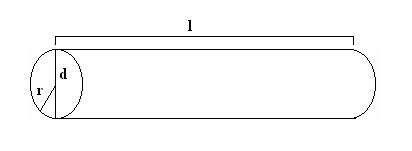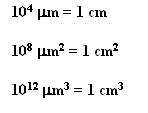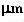Use area/volume calculations and conversion factors to calculate muscle strength

Muscle fibers respond to exercise by hypertrophy, or an increase in size. Since a muscle fiber is shaped roughly like a cylinder, it’s easy to figure out its cross-sectional area or its volume from looking at it on a slide and measuring the diameter. Since a muscle fiber’s contractile strength is usually directly related to its size, you can even make some inferences about the muscle’s strength, just from the information you get by looking at its diameter.

This is an example of a SIMPLIFYING MODEL. A muscle cell would be hard to deal with directly, so we assume it is a simple cylinder. Now we can use the equations for calculating a cylinder's cross-sectional area..Cross-sectional area of a cylinder =If the cylinder above is a muscle fiber, its diameter might be 100 micrometers (100). What would its cross-sectional area be?

A strong human muscle can exert 8 kg of force per square centimeter of muscle cross section. How much force could the muscle cell above exert if it were a strong muscle?

Use your skill with Excel spreadsheets to create a spreadsheet that will do these calculations for you. Here's a possible set of headings:Use your spreadsheet to calculate muscle strength for 10 different muscle diameters and graph them.

Turn in your spreadsheet and graph and a sentence describing the effect increasing muscle size has on muscle strength.